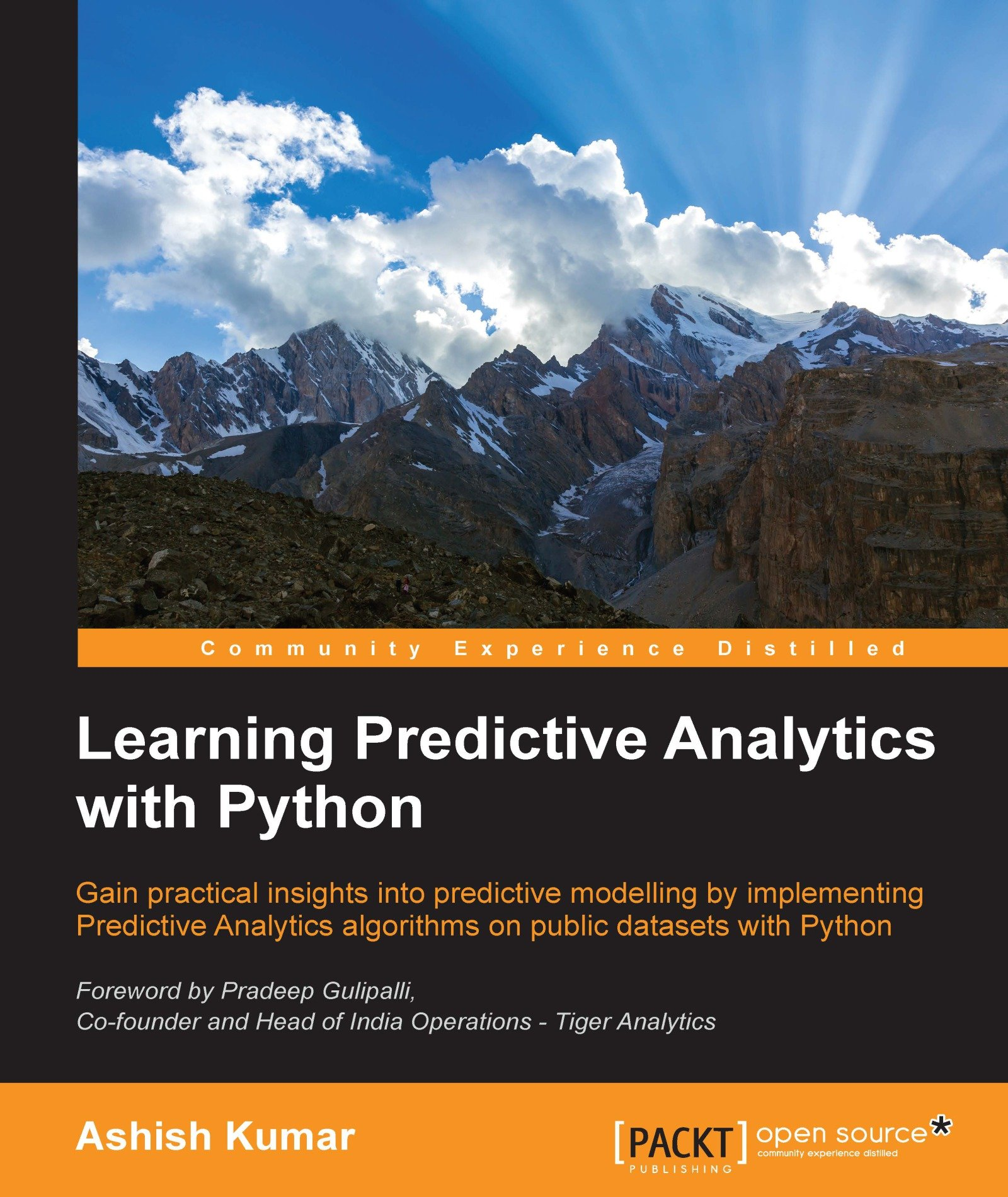### Learning Predictive Analytics with Python

Posted by jack_miller | Published a year agoWith 11 ratings

#### Purchased At: \$39.69 (12 used & new offers)

Gain practical insights into predictive modelling by implementing Predictive Analytics algorithms on public datasets with Python

• A step-by-step guide to predictive modeling including lots of tips, tricks, and best practices
• Get to grips with the basics of Predictive Analytics with Python
• Learn how to use the popular predictive modeling algorithms such as Linear Regression, Decision Trees, Logistic Regression, and Clustering

## Who This Book Is For

If you wish to learn how to implement Predictive Analytics algorithms using Python libraries, then this is the book for you. If you are familiar with coding in Python (or some other programming/statistical/scripting language) but have never used or read about Predictive Analytics algorithms, this book will also help you. The book will be beneficial to and can be read by any Data Science enthusiasts. Some familiarity with Python will be useful to get the most out of this book, but it is certainly not a prerequisite.

## What You Will Learn

• Understand the statistical and mathematical concepts behind Predictive Analytics algorithms and implement Predictive Analytics algorithms using Python libraries
• Analyze the result parameters arising from the implementation of Predictive Analytics algorithms
• Write Python modules/functions from scratch to execute segments or the whole of these algorithms
• Recognize and mitigate various contingencies and issues related to the implementation of Predictive Analytics algorithms
• Get to know various methods of importing, cleaning, sub-setting, merging, joining, concatenating, exploring, grouping, and plotting data with pandas and numpy
• Create dummy datasets and simple mathematical simulations using the Python numpy and pandas libraries
• Understand the best practices while handling datasets in Python and creating predictive models out of them

## In Detail

Social Media and the Internet of Things have resulted in an avalanche of data. Data is powerful but not in its raw form - It needs to be processed and modeled, and Python is one of the most robust tools out there to do so. It has an array of packages for predictive modeling and a suite of IDEs to choose from. Learning to predict who would win, lose, buy, lie, or die with Python is an indispensable skill set to have in this data age.

This book is your guide to getting started with Predictive Analytics using Python. You will see how to process data and make predictive models from it. We balance both statistical and mathematical concepts, and implement them in Python using libraries such as pandas, scikit-learn, and numpy.

You'll start by getting an understanding of the basics of predictive modeling, then you will see how to cleanse your data of impurities and get it ready it for predictive modeling. You will also learn more about the best predictive modeling algorithms such as Linear Regression, Decision Trees, and Logistic Regression. Finally, you will see the best practices in predictive modeling, as well as the different applications of predictive modeling in the modern world.

## Style and approach

All the concepts in this book been explained and illustrated using a dataset, and in a step-by-step manner. The Python code snippet to implement a method or concept is followed by the output, such as charts, dataset heads, pictures, and so on. The statistical concepts are explained in detail wherever required.